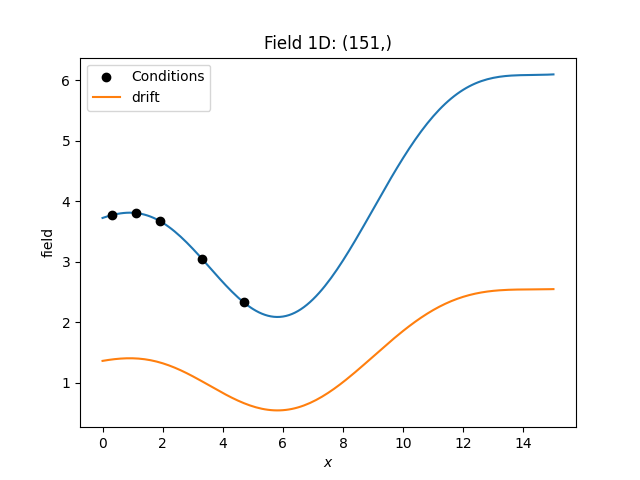# External Drift Kriging¶```import numpy as np
from gstools import SRF, Gaussian, krige

# synthetic condtions with a drift
drift_model = Gaussian(dim=1, len_scale=4)
drift = SRF(drift_model, seed=1010)
cond_pos = [0.3, 1.9, 1.1, 3.3, 4.7]
ext_drift = drift(cond_pos)
cond_val = ext_drift * 2 + 1
# resulting grid
gridx = np.linspace(0.0, 15.0, 151)
grid_drift = drift(gridx)
# kriging
model = Gaussian(dim=1, var=2, len_scale=4)
krig = krige.ExtDrift(model, cond_pos, cond_val, ext_drift)
krig(gridx, ext_drift=grid_drift)
ax = krig.plot()
ax.scatter(cond_pos, cond_val, color="k", zorder=10, label="Conditions")
ax.plot(gridx, grid_drift, label="drift")
ax.legend()
```

Total running time of the script: ( 0 minutes 0.134 seconds)

Gallery generated by Sphinx-Gallery